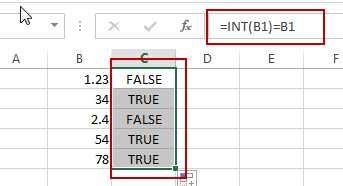# How to Check If a Number is Integer in Excel

This post will guide you how to check if a number is integer in Cells in Excel. How do I test if Cell value is integer with a formula in Excel.

Check If Value is Integer

Assuming that you have a list of data in range B1:B5, in which contain numeric values. And you want to test each cell value if it is an integer, if true, returns TRUE, otherwise, returns FALSE. How can I do it. You can use a formula based on the INT function to achieve the result. Like this:

`=INT(B1)=B1`

Type this formula into a blank cell, such as: Cell C1, and press Enter key on your keyboard. Then copy this formula from cell C1 to range C2:C5 to apply this formula to check values.Let’s see how this formula works:

The INT function try to extract the integer portion from a given cell value, if the returned value is equal to the default cell value, it indicated that numeric value is an integer.

### Related Functions

• Excel INT function
The Excel INT function returns the integer portion of a given number. And it will rounds a given number down to the nearest integer.The syntax of the INT function is as below:= INT (number)…
Related Posts

Cash Denomination Calculator

Every country has different cash denominations, so you may need to calculate the number of different denominations based on the total amount. If it is just a small amount of cash, then you can calculate the different cash denominations manually, ...

Calculate Years Between Dates In Ms Excel

If you are an avid Ms Excel user, then you might have come across a task in which you needed to calculate the years between the dates; you might take it easy and do this task manually, which is also ...

Calculate Number of Hours between Two Times

Calculating the difference between two times might be a valuable statistic for subsequent computations or averages, whether you're producing a time sheet for staff or recording personal exercises. While Excel has a plethora of complex functions, including date and time ...

Extract date from multiple cells containing both date and time

As if you have got a cell containing date along with the time in the same cell and you want to extract the date into a separate cell, then for one or two cells, you can extract the date manually ...

How to Auto Fill Weekdays or Weekends in Excel

Sometimes we may want to enter a sequence of days in excel for some purpose, and we can press Ctrl+; to insert current date into cell, and then drag the cell down to attach following days into other cells, then ...

Sidebar